Documentation

## Estimate the Transfer Function of a Circuit with ADALM1000

This example shows how to use MATLAB to connect to an ADALM1000 source-measurement unit, configure it to generate an arbitrary signal, make live measurements, and use the measurements to calculate the transfer function of the connected circuit.

### Introduction

In this example you have an R-C circuit consisting of a 1 k$\Omega$ resistor in series with a 0.1 $\mu$F capacitor. The R-C circuit is attached to the ADALM1000 device with Channel A of the device providing the voltage stimulus consisting of a chirp signal. The output of Channel A is connected to the resistor, and the ground is connected to the capacitor. Channel B of the device is used to measure the voltage across the capacitor. The following circuit diagram shows the measurement setup.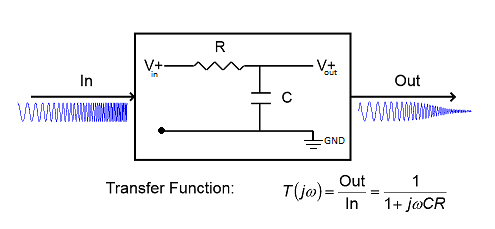You use the knowledge of the stimulus and the measured waveform to estimate the transfer function of the R-C circuit and compare the measured response to the theoretical response of the R-C circuit.

### Discover Devices Connected to Your System

`daq.getDevices`
```ans = adi: Analog Devices Inc. ADALM1000 (Device ID: 'SMU1') Analog input subsystem supports: 0 to +5.0 Volts,-0.20 to +0.20 A ranges Rates from 100000.0 to 100000.0 scans/sec 2 channels ('A','B') 'Voltage','Current' measurement types Analog output subsystem supports: 0 to +5.0 Volts,-0.20 to +0.20 A ranges Rates from 100000.0 to 100000.0 scans/sec 2 channels ('A','B') 'Voltage','Current' measurement types Properties, Methods, Events ```

### Create a Session Using the ADALM1000 Device

`ADISession = daq.createSession('adi')`
```ADISession = Data acquisition session using Analog Devices Inc. hardware: Will run for 1 second (100000 scans) at 100000 scans/second. No channels have been added. Properties, Methods, Events ```

### Add Voltage Source and Measurement Channels

The ADALM1000 device is capable of sourcing and measuring voltage simultaneously on separate channels. Set up the device in this mode.

To source voltage, add an analog output channel with device ID SMU1 and channel ID A, and set its measurement type to Voltage.

`addAnalogOutputChannel(ADISession,'smu1','a','Voltage');`

To measure voltage, add an analog input channel with device ID SMU1 and channel ID B, and set its measurement type to Voltage.

`addAnalogInputChannel(ADISession,'smu1','b','Voltage');`

Confirm the configuration of the session.

`ADISession`
```ADISession = Data acquisition session using Analog Devices Inc. hardware: No data queued. Will run at 100000 scans/second. Number of channels: 2 index Type Device Channel MeasurementType Range Name ----- ---- ------ ------- ------------------- --------------- ---- 1 ao SMU1 A Voltage (SingleEnd) 0 to +5.0 Volts 2 ai SMU1 B Voltage (SingleEnd) 0 to +5.0 Volts Properties, Methods, Events ```

### Define and Visualize a Chirp Waveform for Stimulating the Circuit

Use a chirp waveform of 1 volt amplitude, ranging in frequency from 20 Hz to 20 kHz for stimulating the circuit. The chirp occurs in a period of 1 second.

```Fs = ADISession.Rate; T = 0:1/Fs:1; ExcitationSignal = chirp(T,20,1,20e3,'linear');```

Add a DC offset of 2 V to ensure that the requested output voltage of the device is always above 0 V.

```Offset = 2; ExcitationSignal = ExcitationSignal + Offset;```

Visualize the signal in the time-domain.

```figure(1) plot(T, ExcitationSignal,'Blue') xlim([0 0.15]) xlabel('Time (s)') ylabel('Magnitude (V)') title('Time domain plot of input signal')```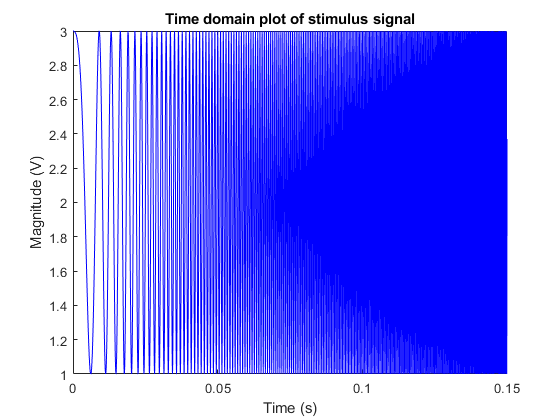Visualize the signal in the frequency domain.

```figure(2) spectrogram(ExcitationSignal,1024,1000,1024,Fs,'yaxis') title('Frequency domain view of the the input signal')```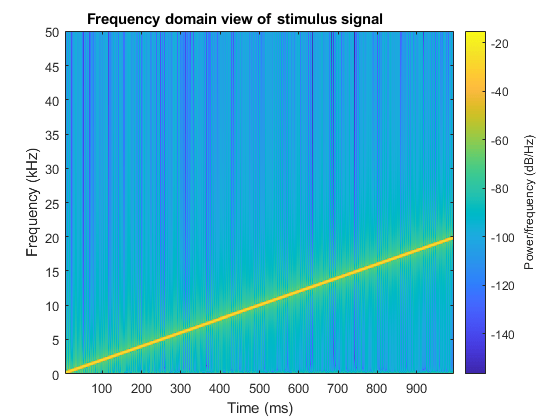### Stimulate the Circuit and Simultaneously Measure the Frequency Response

Queue the data to be sent out by the device on the first channel and measure the voltage across the capacitor on the second channel.

```queueOutputData(ADISession,ExcitationSignal'); MeasuredSignal = startForeground(ADISession);```

### Plot the Stimulus and the Measured Signals

```figure(1) hold on; plot(T, MeasuredSignal,'Red'); xlim([0 0.15]) ylim([1 3]) title('Time domain plot of stimulus and measured signal') legend('Excitation Signal','Measured Signal')```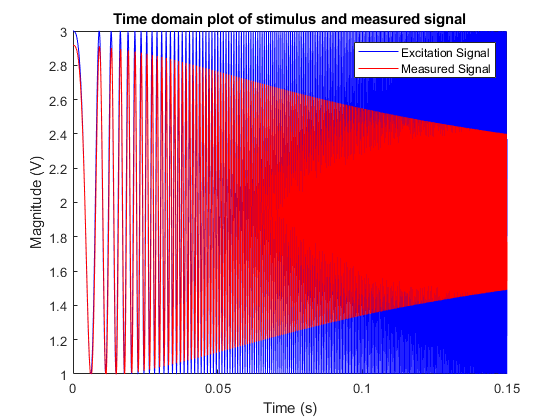```figure(3) spectrogram(MeasuredSignal,1024,1000,1024,Fs,'yaxis') title('Frequency domain view of the the measured signal')```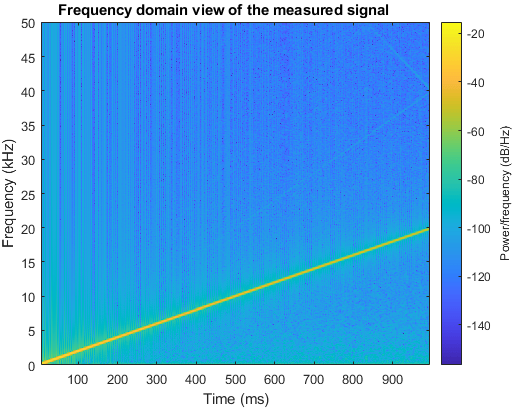### Calculate the Transfer Function of the Circuit

Use comparison of the measured signal and the stimulus signal to calculate the transfer function of the R-C circuit and plot the magnitude response.

Remove DC offset before processing.

```MeasuredSignal = MeasuredSignal - mean(MeasuredSignal); ExcitationSignal = ExcitationSignal - Offset; [TFxy,Freq] = tfestimate(ExcitationSignal, MeasuredSignal,[],[],[],Fs); Mag = abs(TFxy);```

Compare the estimated transfer function to the theoretical magnitude response.

```R = 1000; % Resistance (ohms) C = 0.1e-6; % Capacitance (farads) TFMagTheory = abs(1./(1 + (1i*2*pi*Freq*C*R))); figure(4); semilogy(Freq,TFMagTheory,Freq,Mag); xlim([0 20e3]) xlabel('Frequency (Hz)') ylim([0.05 1.1]) ylabel('Magnitude') grid on legend('Theoretical frequency response','Measured frequency response') title({'Magnitude response of the theoretical'; 'and estimated transfer function'});```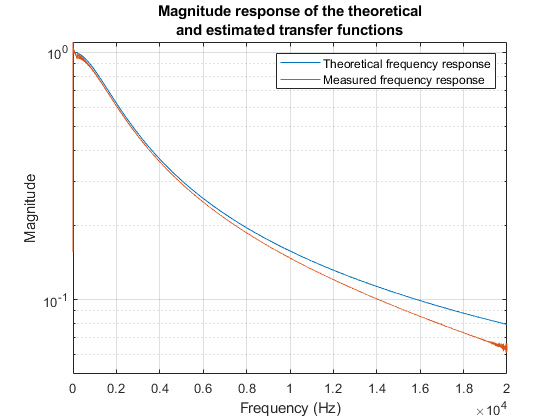### Clear the Session

```clear ADIsession close all```
##### Support평가판 신청